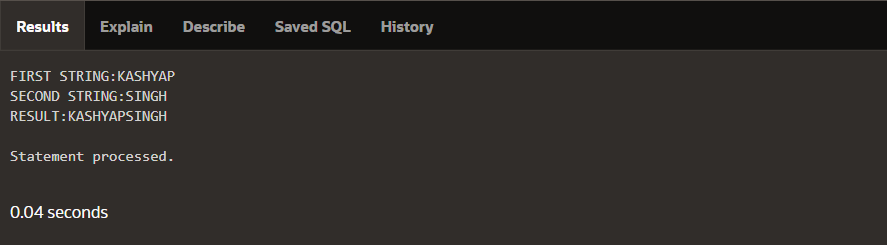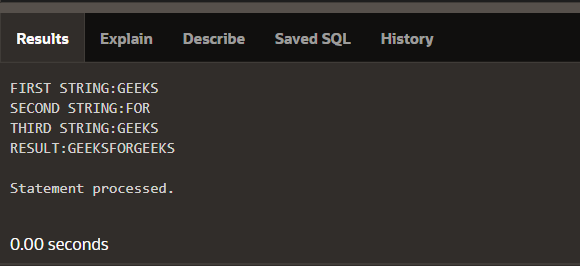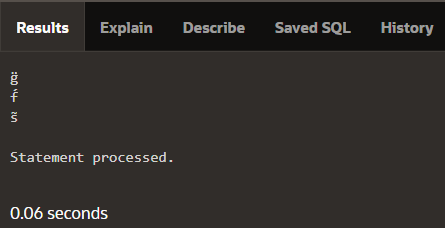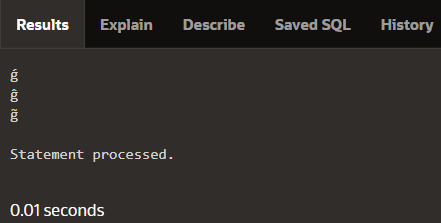# Inbuilt Concat Function in PLSQL

• Difficulty Level : Medium
• Last Updated : 03 Aug, 2021

Prerequisite :  PLSQL BASICS

Introduction :
PLSQL stands for “Procedural Language extensions to SQL” and is used to transform, update and query data in a database. It is grouped into blocks that contain the declaration and statements. And it is integrated with the oracle database (since version 7). And the main idea behind PLSQL is that it adds some programming constraints that are not available in SQL.

1. Concat Function :
Concatenation means joining various strings to form a new string or we can also say that to link something together in the series or in a chain. So in PLSQL, we can use concat() function to join various strings into a single string. So concat() function takes two inputs as a parameter and then it returns the appended string. And we can also use it to concatenate more than two strings and we can see its implementation in the below example.
This function is Supported in Oracle 12c, Oracle 11g, Oracle 10g, Oracle 9i, Oracle 8i.

Example –

```INPUT: STR1='PL' , STR2='SQL'
OUTPUT:PL SQL

INPUT: STR1='KASHYAP' , STR2='SINGH'
OUTPUT:KASHYAP SINGH

INPUT: STR1='GEEKS' , STR2='FOR' , STR3='GEEKS'
OUTPUT:GEEKS FOR GEEKS```

Syntax –

`concat(source1,source2);`

Parameter used –
Here source1 is the first string and source2 is the second string.
The above function will return the appended string.

Example 1 –

```DECLARE
str1 varchar2(25);
str2 varchar2(25);
res varchar2(25);

BEGIN
str1:='KASHYAP';
str2:='SINGH';
res:=concat(str1,str2);

dbms_output.put_line('FIRST STRING:'||str1);
dbms_output.put_line('SECOND STRING:'||str2);
dbms_output.put_line('RESULT:'||res);
END;```

Output –Example  2 –

```DECLARE
str1 varchar2(25);
str2 varchar2(25);
str3 varchar2(25);
res varchar2(25);

BEGIN
str1:='GEEKS';
str2:='FOR';
str3:='GEEKS';
res:=concat(str1,concat(str2,str3);

dbms_output.put_line('FIRST STRING:'||str1);
dbms_output.put_line('SECOND STRING:'||str2);
dbms_output.put_line('THIRD STRING:'||str3);
dbms_output.put_line('RESULT:'||res);
END;```

Output 2 –2. Compose Function :

• In PLSQL the String is actually the sequence of characters with an optional size specification and the character could be a letter, blank, number, special character, or a combination of all. The Compose function basically returns a Unicode string.
• Unicode is a standard for working with a wide range of characters. Each symbol has a code point (a number), and these code points can be encoded (converted to a sequence of bytes) using a variety of encodings.
• UTF-8 is one such encoding. The low code points are encoded using a single byte, and higher code points are encoded as sequences of bytes.

The unistring values that can be combined with other characters in the compose function are:

• unistr(‘\0302’) – circumflex ( ^ )
• unistr(‘\0300’) – grave accent ( ` )
• unistr(‘\0308’) – umlaut ( ¨ )
• unistr(‘\0301’) – acute accent ( ´ )
• unistr(‘\0303’) – tilde ( ~ )

Example –

```INPUT-COMPOSE('o' || unistr('\0308') )
OUTPUT-ö

INPUT-COMPOSE('a' || unistr('\0302') )
OUTPUT-â```

Syntax –

`COMPOSE(STRING)`

Parameter Used –
String – It is the input String whose Unicode string needs to be generated.
The above Function will return the Unicode String.

Example 1 –

```DECLARE
Var1 char:='g';
Var2 char:='f';
Var3 char:='s';

BEGIN
dbms_output.put_line(COMPOSE(Var1 || unistr('\0308' )));
dbms_output.put_line(COMPOSE(Var2 || unistr('\0301' )));
dbms_output.put_line(COMPOSE(Var3 || unistr('\0303' )));
END;  ```

Output –Example 2 –

```DECLARE
Var1 char:='g';
BEGIN
dbms_output.put_line(COMPOSE(Var1 || unistr('\0301' )));
dbms_output.put_line(COMPOSE(Var1 || unistr('\0302' )));
dbms_output.put_line(COMPOSE(Var1 || unistr('\0303' )));
END;  ```

Output –My Personal Notes arrow_drop_up